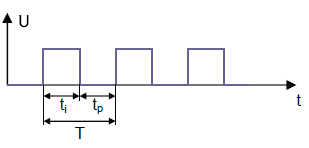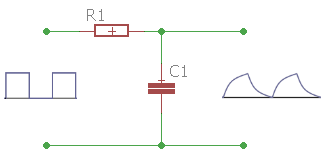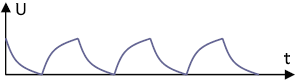# RC integrator, calculate online

Calculator and formulas for calculating an RC integrator

## Calculate RC integrator online

This function can be used to calculate the properties of an RC integrator. The function calculates the capacitor, the resistance or the period or the frequency.

Calculate integrator

 What should be calculated? Resistor Capacitor Frequency / Period Input Resistor mΩ Ω kΩ MΩ Capacitor F mF µF nF pF Frequency Period Pulse Hz kHz MHz GHz pSek nSek µSek mSek Sek pSek nSek µSek mSek Sek Decimal places 0 1 2 3 4 6 8 10 Result Resistor Capacitor Frequency Period

### Formulas for the RC integrator

The integrator works as a pulse shaper stage. The CR element generates a pulse-like alternating voltage at the output of the circuit from a square-wave voltage at the input.T = Period
t1 = PulseIf the length of the rectangular pulse (t1) corresponds to 5 times the time constant Τ of the CR circuit, a pulse train like the one in the figure below is created.$$\displaystyle t1= 5 · R · C$$   ⇒   $$\displaystyle R=\frac{t1}{5 · C}$$   ⇒   $$\displaystyle C=\frac{t1}{5 · R}$$

If the pulse duration of the input voltage is greater than 5 T, steeper pulses equal to the input voltage are generated

### The time constant? (tau)

The time constant of an RC element is the product of R • C. The unit of measurement is the second. The symbol is the Greek letter $$τ$$ (tau),

After 5 tau the charge is approx. 99.3%.